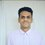# Parity - "A Lethal Weapon"

This note is to show how $$‘PARITY’$$ can be used very effectively to solve olympaid problems .

$'PARITY’$ means that $odd+odd=even,odd+even=odd,even+even=even,odd(odd)=odd,odd(even)=even,even(even)=even$

To explain its significance a example of question of $RMO 2016$

QUESTION : $f(x)=x^{3}-(k-3)x^{2}-11x+(4k-8)$ Find all integers $k$ such that roots of $f(x)$ are integers.

Proof : let $a,b,c$ are integral roots of $f(x)$

This implies $a+b+c=(k-3),ab+bc+ac=-11,abc=4(2-k)$

Since $a,b,c$ are integers and $abc=4(2-k)$ therefore atleast one of them is even or $k=2$ but $k=2$ doesn’t give integral roots but $ab+bc+ac=-11$ therefore at a time 2 or 3 of ${a,b,c}$ can’t be even .

Therefore only one of them is even .

Let $a$ is even .

Also $a+b+c=even;k-3=even;k=odd$

$f(x)=(x-a)(x-b)(x-c)$ , this implies for $x$ be an integer $f(x)$ is also an integer . Now put $x=2$(because at $x=2$ the $k$ term diappeas in $f(x)$) in $f(x)$ this gives $f(2)=(2-a)(2-b)(2-c)=-10$ . Now since $a$ is even therefore $(2-a)={-2,2}$ where $2-a=-2$ is the essential case as $2-a=2$ makes $k=even$.

So $a=4$ is a root of $f(x)$ . after putting values of $x=4$ in $f(x)$ we get $k=5,b=1,c=-3$ which matches our condition that ${k,b,c}=odd$ .Note by Shivam Jadhav
4 years, 8 months ago

This discussion board is a place to discuss our Daily Challenges and the math and science related to those challenges. Explanations are more than just a solution — they should explain the steps and thinking strategies that you used to obtain the solution. Comments should further the discussion of math and science.

When posting on Brilliant:

• Use the emojis to react to an explanation, whether you're congratulating a job well done , or just really confused .
• Ask specific questions about the challenge or the steps in somebody's explanation. Well-posed questions can add a lot to the discussion, but posting "I don't understand!" doesn't help anyone.
• Try to contribute something new to the discussion, whether it is an extension, generalization or other idea related to the challenge.
• Stay on topic — we're all here to learn more about math and science, not to hear about your favorite get-rich-quick scheme or current world events.

MarkdownAppears as
*italics* or _italics_ italics
**bold** or __bold__ bold
- bulleted- list
• bulleted
• list
1. numbered2. list
1. numbered
2. list
Note: you must add a full line of space before and after lists for them to show up correctly
paragraph 1paragraph 2

paragraph 1

paragraph 2

[example link](https://brilliant.org)example link
> This is a quote
This is a quote
    # I indented these lines
# 4 spaces, and now they show
# up as a code block.

print "hello world"
# I indented these lines
# 4 spaces, and now they show
# up as a code block.

print "hello world"
MathAppears as
Remember to wrap math in $$ ... $$ or $ ... $ to ensure proper formatting.
2 \times 3 $2 \times 3$
2^{34} $2^{34}$
a_{i-1} $a_{i-1}$
\frac{2}{3} $\frac{2}{3}$
\sqrt{2} $\sqrt{2}$
\sum_{i=1}^3 $\sum_{i=1}^3$
\sin \theta $\sin \theta$
\boxed{123} $\boxed{123}$

## Comments

Sort by:

Top Newest

Awesome use of parity!

- 4 years, 8 months ago

Log in to reply

Great solution!

- 4 years, 8 months ago

Log in to reply

Why didn't you consider the case 2-a=|10|?

- 4 years, 8 months ago

Log in to reply

ab+bc+ca=-11 not satisfied

- 4 years, 8 months ago

Log in to reply

Yes. Parity is of great help in many cases. It needs a little practice before we can make great use of it. I had used it in silution of quadratic equations. Thank you for the notes.

- 4 years, 1 month ago

Log in to reply

- 4 years, 8 months ago

Log in to reply

- 4 years, 8 months ago

Log in to reply

×

Problem Loading...

Note Loading...

Set Loading...